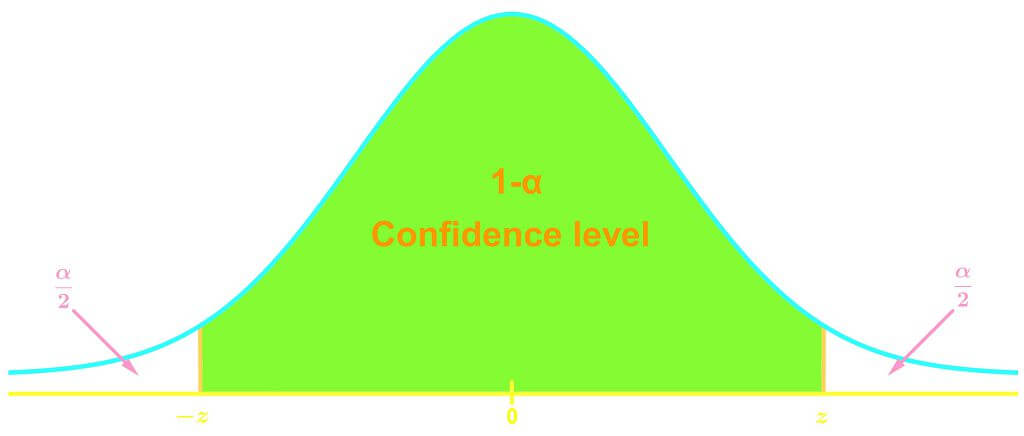# Confidence levels and critical values

### Confidence levels and critical values

#### Lessons

• Introduction
Confidence Level: $1-\alpha$

.Common Confidence levels:
$\cdot$ $0.90$$Z_\frac{\alpha}{2}=1.645$
$\cdot$ $0.95$$Z_\frac{\alpha}{2}=1.96$
$\cdot$ $0.99$$Z_\frac{\alpha}{2}=2.575$
Critical value is the z-score relating to a confidence level

• 1.
Finding the Critical Value
Find the resulting critical values from the following confidence levels:
a)
A confidence level of 0.50

b)
A confidence level of 0.90

c)
A confidence level of 0.95

d)
A confidence level of 0.99

• 2.
What would be the value of $Z_\frac{\alpha}{2}$ for a confidence level of 0.98?

• 3.
Theoretical interpretation of the confidence level and critical value
What would be the resulting critical value for
a)
A confidence level of 1?

b)
A confidence level of 0?